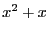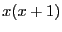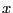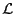Next: Using the ALIAS parser Up: Parser, Generic Solver and Previous: Parser, Generic Solver and   Contents

The ALIAS parser

As it has been seen in the previous chapters the interval evaluation of an equation is highly dependent upon its formulation (for example the evaluation ofmay be quite different from the evaluation offor the same range on). For testing purposes it may therefore be interesting to change the formulation of an equation (for example by using MAPLE), write its analytical form in a file and test what will be the interval evaluation of this equation in this analytical form: this is one objective of the ALIAS parser. Basically this parser takes as input:
• a file, called a formula file, in which is written the analytical form of the equation
• name and ranges for the variables
and will then produces as output the interval evaluation of the equations. In the formula file you may indicate an arbitrary number of equations, each of them being prefixed by eq=. For example

eq=(y^2-1)*z+(2*y*t-2)*x
eq=2+(-10*t+(-10+2*y*t)*y)*y+((4+4*y^2)*z+(4+4*y*t-x*z)*x)*x
eq=(2*y*t-2)*z+(t^2-1)*x
eq=2+(4*x+(4-x*z)*z)*z+((-10+4*z^2)*y+(-10+4*x*z+2*y*t)*t)*t
is a valid formula file in parser format. There is however a limitation on the number of unknowns which cannot exceed 200. Note that the variable names in a formula file may be any name composed with lower and upper cases, integer numbers and underscore, with the constraint that the first character should be a letter. Note also that a MAPLE library enables to produce a formula file directly from MAPLE equations (see section 12.3).

The parser may handle almost any complex analytical equation based on the most classical mathematical functions, using MAPLE notation. Currently you may use the following operators:

• arithmetic operator: "+","-","/","*"," " or "**" (power)
• trigonometric and inverse trigonometric functions: sin, cos, tan, arcsin, arccos, arctan (which may be used with the syntax arctan(x) or arctan(y,x))
• hyperbolic functions: sinh, cosh, tanh, arcsinh, arccosh, arctanh
• mathematical functions: abs (absolute value), log, log10, exp, sqrt
• integer functions: ceil (returns an interval which bounds are the smallest integer greater than or equal to each bound of the box, floor (returns an interval which bounds are the greatest integer less than or equal to each bound of the box), round (returns an interval which bounds are the nearest integer of each bound of the box)
Beside these functions we have added a few more operators:
• Min: this operator takes as input a list of mathematical expression and will return an interval reduced to the minimum value of all the lower bound of the interval evaluation of each term in the list. For example:

Min(3,3*x1,3*x2+x3,4*(x2+x1))

for x1 in [-1,1], x2 in [0,2], x3 in [3,10] will return the interval [-4,-4]
• Max: this operator is similar to Min except it returns the maximal value of the upper bound on the interval evaluation. For the previous example Max will return the interval [16,16]
• MinMax: this operator applied on the listwill return the interval [Min(), Max()]. In the previous example the interval will be [-4,16]

The parser may also handle intervals. For example you may evaluate an equation in which some coefficients are intervals. In the equation these coefficients should be indicated using the MAPLE notation as in the following example:

INTERVAL(0.1 .. sin(1))

SubsectionsNext: Using the ALIAS parser Up: Parser, Generic Solver and Previous: Parser, Generic Solver and   Contents
Jean-Pierre Merlet 2012-12-20